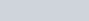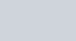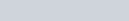Find the equation of the tangent line to the curve
Question:

Find the equation of the tangent line to the curve $y=x^{2}-2 x+7$ which is

(a) parallel to the line 2x − y + 9 = 0

(b) perpendicular to the line 5y − 15x = 13.

Solution:

The equation of the given curve is $y=x^{2}-2 x+7$.

On differentiating with respect to x, we get:

$\frac{d y}{d x}=2 x-2$

(a) The equation of the line is 2x − y + 9 = 0.

2x − y + 9 = 0 ⇒ y = 2+ 9

This is of the form y = mx c.

∴Slope of the line = 2

If a tangent is parallel to the line 2x − y + 9 = 0, then the slope of the tangent is equal to the slope of the line.

Therefore, we have:

2 = 2x − 2

$\Rightarrow 2 x=4$

$\Rightarrow x=2$

Now, x = 2

$\Rightarrow y=4-4+7=7$

Thus, the equation of the tangent passing through (2, 7) is given by,

$y-7=2(x-2)$

$\Rightarrow y-2 x-3=0$

Hence, the equation of the tangent line to the given curve (which is parallel to line 2x − y + 9 = 0) is.

(b) The equation of the line is 5y − 15x = 13.

5y − 15x = 13 ⇒This is of the form y = mx c.

∴Slope of the line = 3

If a tangent is perpendicular to the line $5 y-15 x=13$, then the slope of the tangent is $\frac{-1}{\text { slope of the line }}=\frac{-1}{3}$.

$\Rightarrow 2 x-2=\frac{-1}{3}$

$\Rightarrow 2 x=\frac{-1}{3}+2$

$\Rightarrow 2 x=\frac{5}{3}$

$\Rightarrow x=\frac{5}{6}$

Now, $x=\frac{5}{6}$

$\Rightarrow y=\frac{25}{36}-\frac{10}{6}+7=\frac{25-60+252}{36}=\frac{217}{36}$

Thus, the equation of the tangent passing through $\left(\frac{5}{6}, \frac{217}{36}\right)$ is given by,

$y-\frac{217}{36}=-\frac{1}{3}\left(x-\frac{5}{6}\right)$

$\Rightarrow \frac{36 y-217}{36}=\frac{-1}{18}(6 x-5)$

$\Rightarrow 36 y-217=-2(6 x-5)$

$\Rightarrow 36 y-217=-12 x+10$

$\Rightarrow 36 y+12 x-227=0$

Hence, the equation of the tangent line to the given curve (which is perpendicular to line 5y − 15x = 13) is.